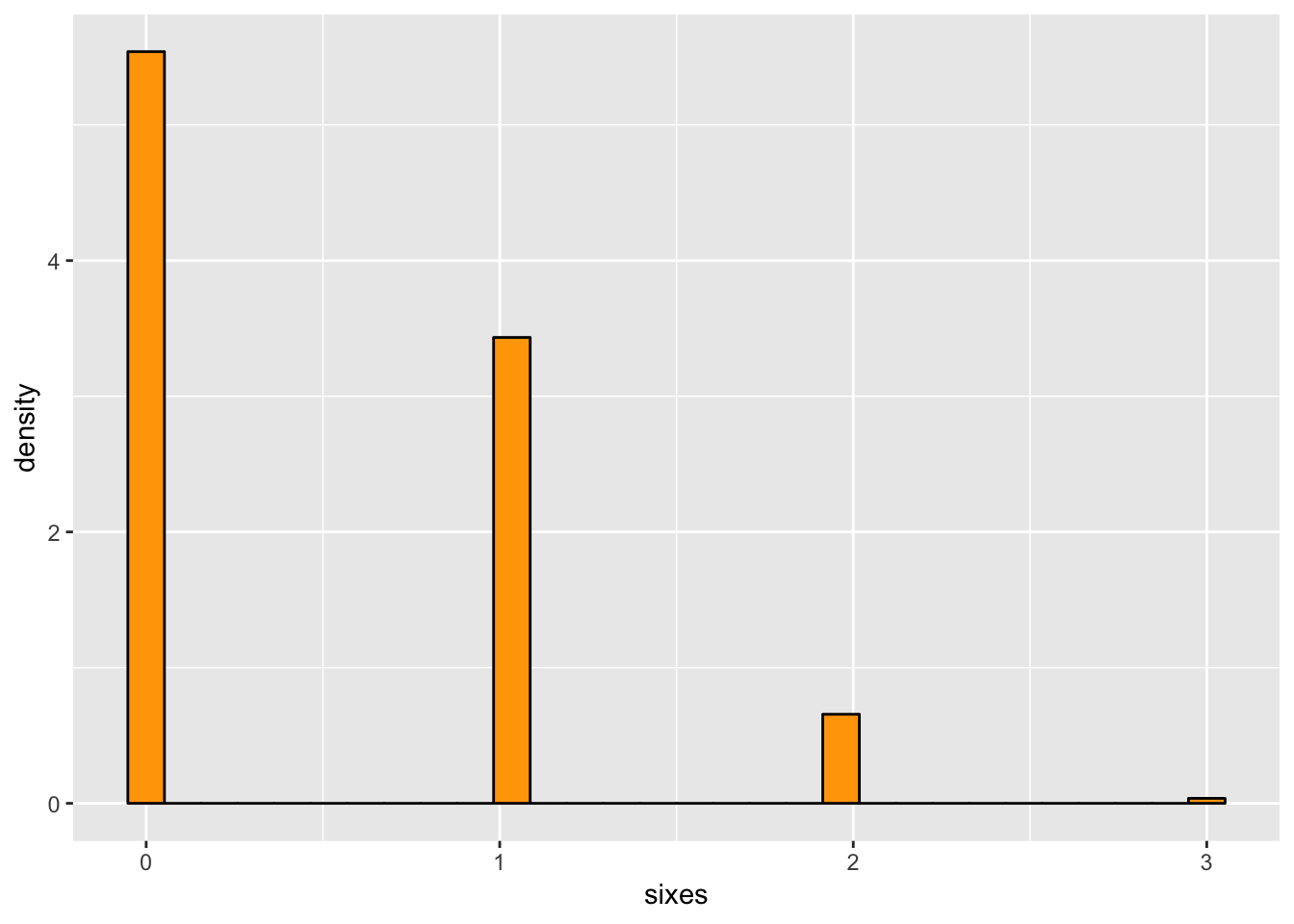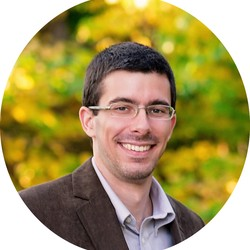# The Power of Simulation

I’m reading a book that deals with the history of probability theory (Classic Problems of Probability by Prakash Gorroochurn). It is interesting to look back from a modern perspective and realize how many heated arguments could have been resolved in a few minutes if the participants would have had access to a computer.

For example, Chapter 1 deals with Cardano and how he (understandably) confused probability with expectation. If you roll a die 3 times, the expectation value of the number of sixes is 0.5, but the probability of one six is 0.347, and the probability of at least one six is 0.421.

Of course, this is easily resolved theoretically with the binomial distribution, but we can also simulate it and gain some intuition in a few lines of R:

library(tidyverse)
# generate 10,000 samples of rolling 3 dice
samples <- rerun(10000, sample(1:6, 3, replace = TRUE))
# count the number of sixes in each sample
sixes <- map_dbl(samples, ~ sum(.x == 6))

# the expectation value
mean(sixes)
##  0.5024
# the probability of one six
mean(sixes == 1)
##  0.3552
# the probability of at least one six
mean(sixes >= 1)
##  0.4269

These values aren’t exactly the same as the theoretical values, but we can definitely tell that there is a difference between the expectation and the probability. We can also plot the results to get an idea of the distribution:

ggplot() +
geom_histogram(aes(x = sixes, y = ..density..),
fill = "orange", color = "black")
## Warning: list_len() is deprecated as of rlang 0.2.0.
## Please use new_list() instead.
## This warning is displayed once per session.It is hard to see on the histogram, but there are a few throws where we get 3 sixes:

sum(sixes == 3)
##  38

This happens with a simulated probability of 0.0038. The theoretical value is $\left( \frac{1}{6} \right)^3 = 0.00462963.$

In short, our current access to computers gives us a huge advantage over the early probabilists. It almost seems unfair. We would likely make a lot of the same mistakes they did with the theory, but for many problems we can quickly and easily check whether or not our theoretical calculations are correct by comparing with a simulation.##### Landon Lehman
###### Data Scientist

My research interests include data science, statistics, physics, and applied math.